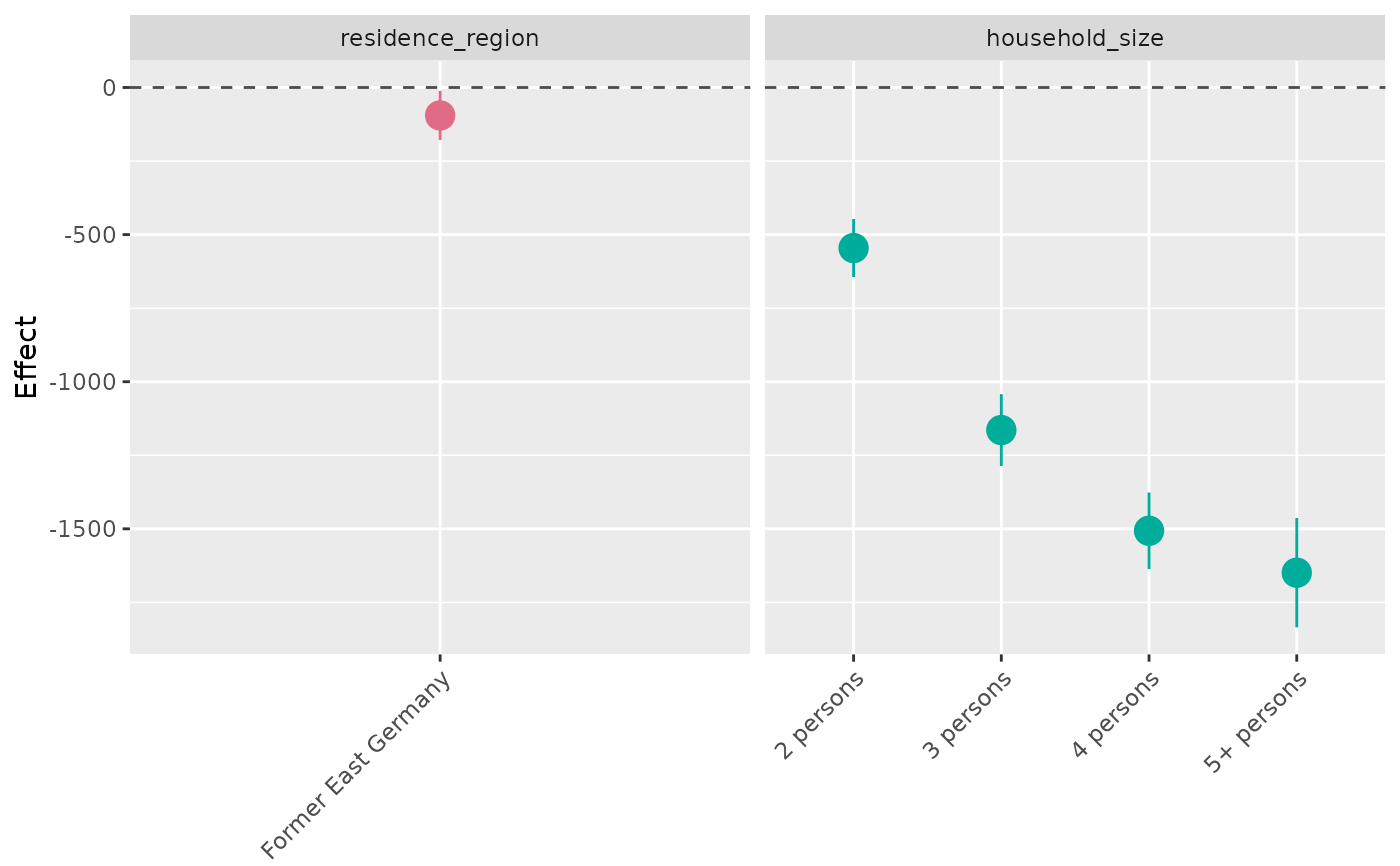Create an effect plot of linear effects of a model fitted with gam or bam.

## Usage

plot_linearEffects(
model,
variables = NULL,
return_plotData = FALSE,
refCat = FALSE,
...
)

## Arguments

model

Model fitted with gam or bam.

variables

Optional character vector of variable names specifying which effects should be plotted. The order of the vector corresponds to the order in the effect plot. If the argument is not specified, all linear effects are plotted according to the order of their appearance in the model output.

return_plotData

If TRUE, the dataset prepared for plotting is returned. Defaults to FALSE.

refCat

If TRUE, reference categories are added to the output for categorical covariates. Defaults to FALSE.

...

Additional arguments passed to extract_summary_linearEffects.

ggplot object

## Details

If the model was estimated with a log or logit link, the function automatically performs an exponential transformation of the effect.

## Author

Alexander Bauer alexander.bauer@stat.uni-muenchen.de

## Examples

library(APCtools)
library(mgcv)

data(travel)
model <- gam(mainTrip_distance ~ te(age, period) + residence_region +
household_size + s(household_income), data = travel)

plot_linearEffects(model)